#Interactive Real Analysis

Next | Previous | Glossary | Map

## 2. Infinity and Induction

### 2.5. Pi and e are irrational

Pi and e (Euler's number) are two numbers that occur everywhere in mathematics. Both are irrational and in fact transcendental numbers. In this little appendix we will prove that both numbers are irrational. We will not show that they are also transcendental numbers. The proofs will actually use tools from much later sections, but they might be interesting nevertheless, and the results fit well in this section.

First will now prove that e (Euler's number) is irrational. In fact, it is a transcendental number, but that requires a lot of work to prove.

 Theorem 2.5.3: e is irrational Euler's number e is irrational

Proof: We know that for any integer n(check the definition of Euler's number and the definition of Euler' series) where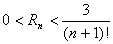Now suppose that e was rational, i.e. there are two positive integers a and b with e = a / b.

Choose n > b. Then, using the above representation, we have: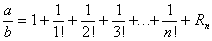or multiplying both sides by n! we get:Since n > b, the left side of this equation represents an integer, and hence n! Rn is also an integer. But we know thatso that if n is large enough the left side is less than 1. But then n! Rn must be a positive integer less than 1, which is a contradiction.Next we prove that Pi is irrational, which is a lot harder to do. We need a preliminary result:

 Lemma 2.5.1: Define a function fn(x) =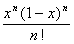. Then this function has the following properties: 0 < fn(x) < 1 / n! for 0 < x < 1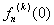and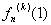are both integers

Proof: Using the binomial theorem, we see that when the numerator of the function is multiplied out, the lowest power of x will be n, and the highest power is 2n. Therefore, the function can be written as

fn(x) =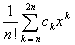where all coefficients are integers. It is clear from this expression that= 0 for k < n and for k > 2n.

Also, looking at the sum more carefully, we see that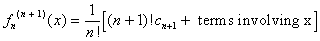...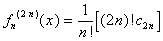But this implies thatis an integer for any k.

Moreover, sincewe also have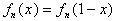Thereforeis an integer for any k.
[ x ]
Also note that |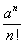| <for n large enough ( n > 2a) and any a. Now we can prove the result of this chapter:
 Theorem 2.5.2: Pi is irrational Pi is irrational

Proof: We will prove that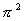is not rational, which implies the assertion. Suppose it was rational. Then there are two positive integers a and b with= a / b
Define the function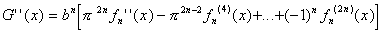Each of the factors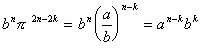is an integer, by assumption on. We also know thatandare integers as well. Hence, G(0) and G(1) are both integers.

Differentiating G(x) twice, we get: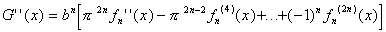The last term in this equation, the (2n+2)-th derivative, is zero, by the properties of the function f. Adding G(x) and G''(x) we get: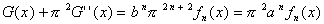Now define another function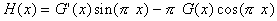Then, using the above formula for G(x) +G''(x), we have: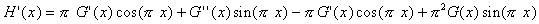=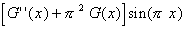=Now we can use the second fundamental theorem of calculus to conclude:= H(1) - H(0)
==[ G(1) + G(0)]
Thus, the integralis an integer. But we also know that 0 < fn(x) < 1 / n! for 0 < x < 1. Therefore, estimating the above integral, we get
0 <<for 0 < x < 1. Therefore, we can estimate our integral to get
0 <<< 1
Here we have used the fact that the last fraction approaches zero if n is large enough. But now we have a contradiction, because that integral was supposed to be an integer. Since there is no positive integer less than 1, our assumption thatwas rational resulted in a contradiction. Hence,must be irrational.This prove is, admittedly, rather curious. Aside from the assumption that Pi is rational (leading to the contradiction) the only other properties of Pi that are really involved in this proof are that

sin() = 0, cos() = 1
and we need the defining properties of the trig. functions that
sin'(x) = cos(x), cos'(x) = - sin(x), sin(0) = 0, cos(0) = 1
That does not make the proof much clearer, but it illustrates that some essential properties ofare indeed used along the way, and this proof will not work for any other number.

Taken from Calculus (2nd Edition), by Michael Spivak
Publish or Perish, Inc, 1980, pages 307 - 310